### Home > AC > Chapter 16 > Lesson 16.9.2.2 > Problem9-82

9-82.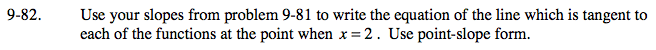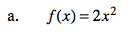From 9-81, m = 8,f(2) = (2)2² = 8
Equation of line: y − 8 = 8(x − 2)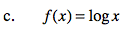From 9-81, m = 0.217, f(2) = log (2) = 0.301
Equation of line: y − 0.301 = 0.217(x − 2)
y = 0.217(x − 2) + 0.301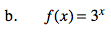From 9-81, m = 9.888, y − 9 = 9.888(x − 2)
Equation of line: y = 9.888(x − 2) + 9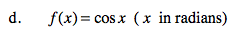From 9-81, m = −0.909
f(2) = cos(2) = −0.416
Equation of line: y − (−0.416) = −0.909(x − 2)
y = −0.909(x − 2) − 0.416